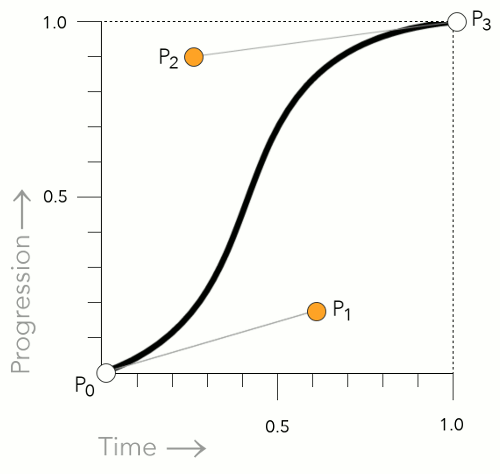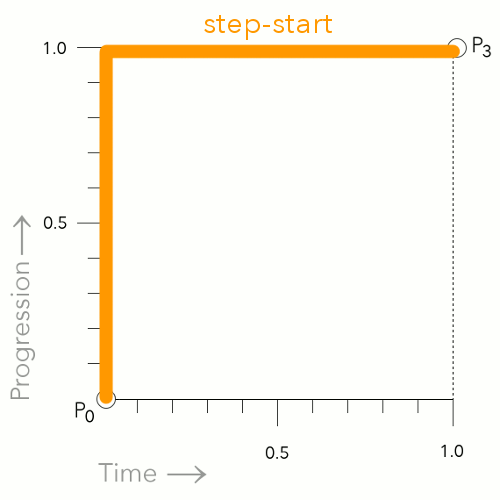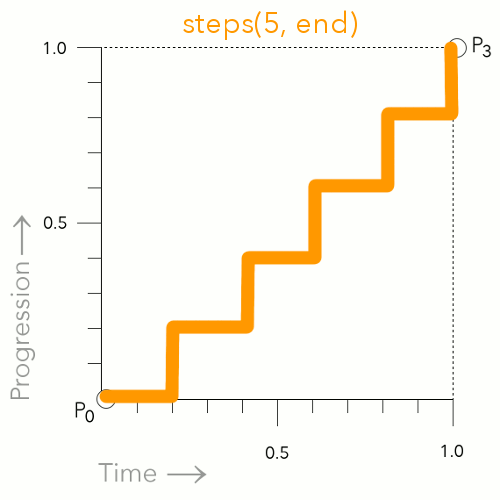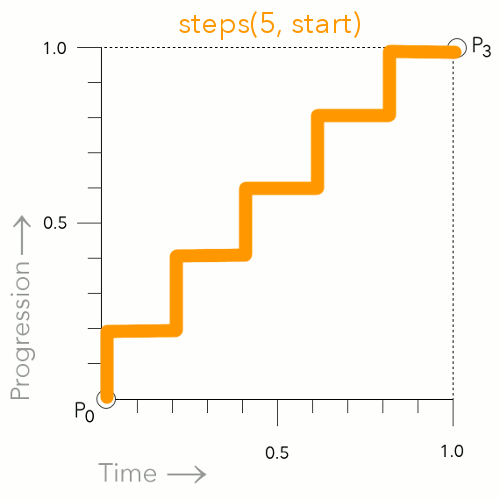# CSS <single-transition-timing-function> Data Type

The `<single-transition-timing-function>` data type is used in transitions and animations to determine how values change between the start and end points.

The `<single-transition-timing-function>` type denotes a timing function that can be used with the `transition-timing-function` and `animation-timing-function` properties. It enables you to create an acceleration curve, so that the speed of the animation can vary over its duration.

For example, if your animation moves an element from left to right, you could have it start off slowly, then increase its speed until it races to the finish point. Or you could do the opposite — start it fast then let it slow down to the finish point. Or you could do a combination.

## Possible Values

The official syntax for the `<single-transition-timing-function>` data type is:

So when you see `<single-transition-timing-function>` (including the `<` and `>`) anywhere in the CSS specifications, this refers to the fact that the value can be one of the valid timing functions specified by CSS.

These timing functions are as follows:

ease
The ease function is equivalent to `cubic-bezier(0.25, 0.1, 0.25, 1.0)`.
linear
The linear function is equivalent to `cubic-bezier(0.0, 0.0, 1.0, 1.0)`.
ease-in
The ease-in function is equivalent to `cubic-bezier(0.42, 0, 1.0, 1.0)`.
ease-out
The ease-out function is equivalent to `cubic-bezier(0, 0, 0.58, 1.0)`.
ease-in-out
The ease-in-out function is equivalent to `cubic-bezier(0.42, 0, 0.58, 1.0)`.
`cubic-bezier()`
Specifies a cubic Bézier curve. The four values specify points P1 and P2 of the curve as (x1, y1, x2, y2). Both x values must be in the range [0, 1], but the y values can exceed that range.
step-start
Represents the timing function `steps(1, start)`. This keyword specifies that the animation will jump immediately to the end state and stay in that position until the end of the animation.
step-end
Represents the timing function `steps(1, end)`. The animation stays in its initial state until the end, where it directly jumps to its final position.
steps([, [ start | end ] ]?)
Positive integer representing the amount of equidistant treads composing the stepping function. For example, `steps(5, end)` indicates that there are 5 treads, with the last one occuring right before the end of the animation.

## Examples

Here are some examples of the same animation but using different timing functions. Here we use the `transition-timing-function` property to specify the timing function. Like this:

Hover over the orange box in each of the following examples. You'll see how each one progresses throughout the animation slightly differently (as defined by its timing function). Although each animation is the same length (2 seconds long), the speed at which the orange element increases its width varies at different points, depending on the timing function being used.

### The `linear` Timing Function

This example uses the `linear` timing function. This is the equivalent of using `cubic-bezier(0, 0, 1, 1)`.

### The `ease` Timing Function

This example uses the `ease` timing function. This is the equivalent of using `cubic-bezier(0.25, 0.1, 0.25, 1.0)`.

### The `ease-in` Timing Function

This example uses the `ease-in` timing function. This is the equivalent of using `cubic-bezier(0.42, 0, 1.0, 1.0)`.

### The `ease-out` Timing Function

This example uses the `ease-out` timing function. This is the equivalent of using `cubic-bezier(0, 0, 0.58, 1.0)`.

### The `ease-in-out` Timing Function

This example uses the `ease-in-out` timing function. This is the equivalent of using `cubic-bezier(0.42, 0, 0.58, 1.0)`.

### The `step-start` Timing Function

This example uses the `step-start` timing function. This is the equivalent of using `steps(1, start)`. This keyword specifies that the animation will jump immediately to the end state and stay in that position until the end of the animation.

### The `step-end` Timing Function

This example uses the `step-end` timing function. This is the equivalent of using `steps(1, end)`. The animation stays in its initial state until the end, where it directly jumps to its final position.

### The `steps()` Timing Function

This example uses the `steps()` timing function. Here we use `steps(5, end)` which indicates that there are 5 treads, with the last one occuring right before the end of the animation.

### The `cubic-bezier()` Timing Function

This example uses the `cubic-bezier()` function. This function can be handy in cases where none of the other timing functions are suitable, as it allows us to set our own custom Bézier curve.

## How do Cubic Bézier Curves work?

The `ease`, `ease-in`, `ease-out`, `ease-in-out`, `linear`, and `cubic-bezier()` timing functions use a Bézier curve.

For example, the following two lines do the same thing:

So in that case, you could use either the `ease-in` or the `cubic-bezier()` function.

A cubic Bézier curve is defined by four control points, P0, P1, P2, and P3 as shown in the following diagram.A cubic Bézier curve showing the Bézier timing function control points.

The P0 and P3 control points are always set to (0,0) and (1,1). In other words, they don't move.

However, P1 and P2 can be moved with the Bézier curve timing functions. When using the `cubic-bezier()` function, you can specify the location of these two control points by using an x and y value. Like this: `x1, y1, x2, y2`. When using one of the keywords, the keyword uses a predefined set of values (as listed above).

## Step Timing Functions

The `step-end`, `step-start`, and `steps()` functions are all step timing functions. The way these work is that they progress the animation in steps — as opposed to a smooth curve like the Bézier curve functions.

Here's more detail on how they work.

### Using the `step-end` KeywordThe `step-end` keyword causes the animation to remain in the start position until the very end. At first, this may appear as though the animation isn't actually working, as the effect won't happen until the end.

### Using the `step-start` KeywordThe `step-start` keyword causes the animation to jump straight to the end position, then remain there until the end of the animation.

### Using the `steps()` Function

#### `steps(5, end)`This is an example of using `steps(5, end)`.This breaks the animation up into 5 distinct points. The animation waits, then jumps to the next point at the applicable time (which is determined by how many steps you specify).

#### `steps(5, start)`This is an example of using `steps(5, start)`. This is similar to `steps(5, end)`, however, it jumps to the first step immediately instead of waiting for the first time increment.

## CSS Specifications

• The `<single-transition-timing-function>` data type is defined in CSS Transitions (W3C Working Draft)